The Australian Journal of Mathematical Analysis and Applications

 Home News Editors Volumes RGMIA Subscriptions Authors Contact

ISSN 1449-5910

You searched for kim
Total of 158 results found in site

17: Paper Source PDF document

Paper's Title:

The Superstability of the Pexider Type Trigonometric Functional Equation

Author(s):

Gwang Hui Kim and Young Whan Lee

Department of Mathematics, Kangnam
University Yongin, Gyeonggi, 446-702, Korea.
ghkim@kangnam.ac.kr

Department of Computer and Information Security
Daejeon University, Daejeon 300-716, Korea.
ywlee@dju.ac.kr

Abstract:

The aim of this paper is to investigate the stability problem for
the Pexider type (hyperbolic) trigonometric functional equation
f(x+y)+f(x+σy)=λg(x)h(y) under the conditions :
|f(x+y)+f(x+σy)- λg(x)h(y)|≤φ(x),
φ(y)
, and min {φ(x), φ (y)}.

As a consequence, we have generalized the results of stability for
the cosine(d'Alembert), sine, and the Wilson functional equations by J.
Baker, P. Găvruta, R. Badora and R. Ger, Pl.~Kannappan, and G.
H. Kim

10: Paper Source PDF document

Paper's Title:

A Stability of the G-type Functional Equation

Author(s):

Gwang Hui Kim

Department of Mathematics, Kangnam University
Suwon 449-702, Korea.
ghkim@kangnam.ac.kr

Abstract:

We will investigate the stability in the sense of Găvruţă for the G-type functional equation f(φ(x))=Γ(x)f(x)+ψ(x) and the stability in the sense of Ger for the functional equation of the form f(φ(x))=Γ(x)f(x). As a consequence, we obtain a stability results for G-function equation.

10: Paper Source PDF document

Paper's Title:

Evaluation of a New Class of Double Integrals Involving Generalized Hypergeometric Function 4F3

Author(s):

Joohyung Kim, Insuk Kim and Harsh V. Harsh

Department of Mathematics Education,
Wonkwang University, Iksan, 570-749,
Korea.
E-mail: joohyung@wku.ac.kr

Department of Mathematics Education,
Wonkwang University, Iksan, 570-749,
Korea.
E-mail: iki@wku.ac.kr

Department of Mathematics, Amity School of Eng. and Tech.,
Amity University Rajasthan
NH-11C, Jaipur-303002, Rajasthan,
India.
E-mail: harshvardhanharsh@gmail.com

Abstract:

Very recently, Kim evaluated some double integrals involving a generalized hypergeometric function 3F2 with the help of generalization of Edwards's well-known double integral due to Kim,  et al. and generalized classical Watson's summation theorem obtained earlier by Lavoie,  et al. In this research paper we evaluate one hundred double integrals involving generalized hypergeometric function 4F3 in the form of four master formulas (25 each) viz. in the most general form for any integer. Some interesting results have also be obtained as special cases of our main findings.

9: Paper Source PDF document

Paper's Title:

An Existence of the Solution to Neutral Stochastic Functional Differential Equations Under the Holder Condition

Author(s):

Young-Ho Kim

Department of Mathematics,
Changwon National University,
Changwon, Gyeongsangnam-do 51140,
Korea.

E-mail: iyhkim@changwon.ac.kr

Abstract:

In this paper, we show the existence and uniqueness of solution of the neutral stochastic functional differential equations under weakened H\"{o}lder condition, a weakened linear growth condition, and a contractive condition. Furthermore, in order to obtain the existence of a solution to the equation we used the Picard sequence.

8: Paper Source PDF document

Paper's Title:

Viability Theory And Differential Lanchester Type Models For Combat.
Differential Systems.

Author(s):

G. Isac and A. Gosselin

Department Of Mathematics, Royal Military College Of Canada,
P.O. Box 17000, S
tn Forces, Kingston, Ontario, Canada K7k 7b4

URL
:

URL
:

Abstract:

In 1914, F.W. Lanchester proposed several mathematical models based on differential equations to describe combat situations . Since then, his work has been extensively modified to represent a variety of competitions including entire wars. Differential Lanchester type models have been studied from many angles by many authors in hundreds of papers and reports. Lanchester type models are used in the planning of optimal strategies, supply and tactics. In this paper, we will show how these models can be studied from a viability theory stand point. We will introduce the notion of winning cone and show that it is a viable cone for these models. In the last part of our paper we will use the viability theory of differential equations to study Lanchester type models from the optimal theory point of view.

8: Paper Source PDF document

Paper's Title:

On an extension of Edwards's double integral with applications

Author(s):

I. Kim, S. Jun, Y. Vyas and A. K. Rathie

Department of Mathematics Education,
Wonkwang University,
Iksan, 570-749,
Republic of Korea.

General Education Institute,
Konkuk University,
Chungju 380-701,
Republic of Korea.

Department of Mathematics, School of Engineering,
Bhatewar, Udaipur, 313601, Rajasthan State,
India.

Department of Mathematics,
Vedant College of Engineering and Technology,
(Rajasthan Technical University),
Bundi-323021, Rajasthan,
India.

E-mail: iki@wku.ac.kr sjun@kku.ac.kr
yashoverdhan.vyas@spsu.ac.in arjunkumarrathie@gmail.com

Abstract:

The aim of this note is to provide an extension of the well known and useful Edwards's double integral. As an application, new class of twelve double integrals involving hypergeometric function have been evaluated in terms of gamma function. The results are established with the help of classical summation theorems for the series 3F2 due to Watson, Dixon and Whipple. Several new and interesting integrals have also been obtained from our main findings.

6: Paper Source PDF document

Paper's Title:

Fractional exp(-φ(ξ))- Expansion Method and its Application to Space--Time Nonlinear Fractional Equations

Author(s):

A. A. Moussa and L. A. Alhakim

Department of Management Information System and Production Management,
College of Business and Economics, Qassim University,
P.O. BOX 6666, Buraidah: 51452,
Saudi Arabia.
E-mail: Alaamath81@gmail.com

Department of Management Information System and Production Management,
College of Business and Economics, Qassim University,
P.O. BOX 6666, Buraidah: 51452,
Saudi Arabia.
E-mail: Lama2736@gmail.com

Abstract:

In this paper, we mainly suggest a new method that depends on the fractional derivative proposed by Katugampola for solving nonlinear fractional partial differential equations. Using this method, we obtained numerous useful and surprising solutions for the space--time fractional nonlinear Whitham--Broer--Kaup equations and space--time fractional generalized nonlinear Hirota--Satsuma coupled KdV equations. The solutions obtained varied between hyperbolic, trigonometric, and rational functions, and we hope those interested in the real-life applications of the previous two equations will find this approach useful.

4: Paper Source PDF document

Paper's Title:

On the Hohov Convolution Of The Class Sp(α,β)

Author(s):

T. N. Shanmugam and S. Sivasubramanian

Department of Mathematics,
Anna University,
Chennai 600025,
shan@annauniv.edu

Department of Mathematics,
Easwari Engineering College,
Chennai-600089,
sivasaisastha@rediffmail.com

Abstract:

Let F(a,b;c;z) be the Gaussian hypergeometric function and Ia,b;c(f)=zF(a,b;c;z)*f(z) be the Hohlov operator defined on the class A of all normalized analytic functions. We determine conditions on the parameters a,b,c such that Ia,b;c(f) will be in the class of parabolic starlike functions Sp(α,β). Our results extend several earlier results.

4: Paper Source PDF document

Paper's Title:

Komatu Integral Transforms of Analytic Functions Subordinate to Convex Functions

Author(s):

T. N. Shanmugam and C. Ramachandran

Department of Mathematics, College of Engineering,
India
shan@annauniv.edu

Department of Mathematics, College of Engineering,
India
crjsp2004@yahoo.com

Abstract:

In this paper, we consider the class A of the functions f(z) of the form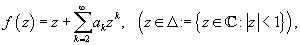which are analytic in an open disk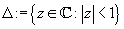and study certain subclass of the class A, for which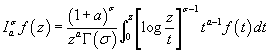has some property. Certain inclusion and the closure properties like convolution with convex univalent function etc. are studied.

4: Paper Source PDF document

Paper's Title:

Approximation of an AQCQ-Functional Equation and its Applications

Author(s):

Choonkil Park and Jung Rye Lee

Department of Mathematics,
Research Institute for Natural Sciences,
Hanyang University, Seoul 133-791,
Korea;

Department of Mathematics,
Daejin University,
Kyeonggi 487-711,
Korea

baak@hanyang.ac.kr
jrlee@daejin.ac.kr

Abstract:

This paper is a survey on the generalized Hyers-Ulam stability of an AQCQ-functional equation in several spaces. Its content is divided into the following sections:

1. Introduction and preliminaries.

2. Generalized Hyers-Ulam stability of an AQCQ-functional equation in Banach spaces: direct method.

3. Generalized Hyers-Ulam stability of an AQCQ-functional equation in Banach spaces: fixed point method.

4. Generalized Hyers-Ulam stability of an AQCQ-functional equation in random Banach spaces: direct method.

5. Generalized Hyers-Ulam stability of an AQCQ-functional equation in random Banach spaces: fixed point method.

6. Generalized Hyers-Ulam stability of an AQCQ-functional equation in non-Archi-medean Banach spaces: direct method.

7. Generalized Hyers-Ulam stability of an AQCQ-functional equation in non-Archi-medean Banach spaces: fixed point method.

4: Paper Source PDF document

Paper's Title:

On a New Class of Eulerian's Type Integrals Involving Generalized Hypergeometric Functions

Author(s):

Sungtae Jun, Insuk Kim and Arjun K. Rathie

General Education Institute,
Konkuk University, Chungju 380-701,
Republic of Korea.

Department of Mathematics Education,
Wonkwang University, Iksan, 570-749,
Republic of Korea.

Department of Mathematics,
Vedant College of Engineering and Technology (Rajasthan Technical University),
Bundi-323021, Rajasthan,
India.

E-mail: sjun@kku.ac.kr, iki@wku.ac.kr, arjunkumarrathie@gmail.com

Abstract:

Very recently Masjed-Jamei and Koepf established interesting and useful generalizations of various classical summation theorems for the 2F1, 3F2, 4F3, 5F4 and 6F5 generalized hypergeometric series. The main aim of this paper is to establish eleven Eulerian's type integrals involving generalized hypergeometric functions by employing these theorems. Several special cases have also been given.

3: Paper Source PDF document

Paper's Title:

Norm Estimates for the Difference between Bochner’s Integral and the Convex Combination of Function’s Values

Author(s):

P. Cerone, Y.J. Cho, S.S. Dragomir, J.K. Kim, and S.S. Kim

School of Computer Science and Mathematics,
Victoria University of Technology,
Po Box 14428, Mcmc 8001, Victoria, Australia.

URL
:

Department of Mathematics Education, College of Education,
Gyeongsang National University, Chinju 660-701, Korea

School of Computer Science and Mathematics,
Victoria University of Technology,
Po Box 14428, Mcmc 8001, Victoria, Australia.

URL
:

Department of Mathematics, Kyungnam University,
Masan,, Kyungnam 631-701, Korea

Department of Mathematics, Dongeui University,
Pusan 614-714, Korea

Abstract:

Norm estimates are developed between the Bochner integral of a vector-valued function in Banach spaces having the Radon-Nikodym property and the convex combination of function values taken on a division of the interval [a, b].

3: Paper Source PDF document

Paper's Title:

Algebraic Approach to the Fractional Derivatives

Author(s):

Institute of Mathematics,
St. Cyril and Methodius University,
P.O. Box 162, 1000 Skopje,
Macedonia
kostatre@iunona.pmf.ukim.edu.mk

tomovski@iunona.pmf.ukim.edu.mk

URL:http://www.pmf.ukim.edu.mk

Abstract:

In this paper we introduce an alternative definition of the fractional derivatives and also a characteristic class of so called ideal functions, which admit arbitrary fractional derivatives (also integrals). Further some ideal functions are found, which lead to representations of the Bernoulli and Euler numbers Bk and Ek for any real number k, via fractional derivatives of some functions at x=0.

3: Paper Source PDF document

Paper's Title:

Stability of a Mixed Additive, Quadratic and Cubic Functional Equation In Quasi-Banach Spaces

Author(s):

Department of Mathematics, Faculty of Sciences,
University of Mohaghegh Ardabili, Ardabil,
Iran
a.nejati@yahoo.com

Faculty of Mathematical Sciences,
University of Tabriz, Tabriz,
Iran

Abstract:

In this paper we establish the general solution of a mixed additive, quadratic and cubic functional equation and investigate the Hyers--Ulam--Rassias stability of this equation in quasi-Banach spaces. The concept of Hyers-Ulam-Rassias stability originated from Th. M. Rassias' stability theorem that appeared in his paper: On the stability of the linear mapping in Banach spaces, Proc. Amer. Math. Soc. 72 (1978), 297--300.

3: Paper Source PDF document

Paper's Title:

On the Generalized Stability and Asymptotic Behavior of Quadratic Mappings

Author(s):

Hark-Mahn Kim, Sang-Baek Lee and Eunyoung Son

Department of Mathematics
Chungnam National University
Daejeon, 305-764,
Republic of Korea

hmkim@cnu.ac.kr

Abstract:

We extend the stability of quadratic mappings to the stability of general quadratic mappings with several variables, and then obtain an improved asymptotic property of quadratic mappings on restricted domains.

3: Paper Source PDF document

Paper's Title:

Shape Diagrams for 2D Compact Sets - Part III: Convexity Discrimination for Analytic and Discretized Simply Connected Sets.

Author(s):

S. Rivollier, J. Debayle and J.-C. Pinoli

Ecole Nationale Supérieure des Mines de Saint-Etienne,
CIS - LPMG, UMR CNRS 5148, 158 cours Fauriel,
42023 Saint-Etienne Cedex 2, France.

Abstract:

Shape diagrams are representations in the Euclidean plane introduced to study 3-dimensional and 2-dimensional compact convex sets. However, they can also been applied to more general compact sets than compact convex sets. A compact set is represented by a point within a shape diagram whose coordinates are morphometrical functionals defined as normalized ratios of geometrical functionals. Classically, the geometrical functionals are the area, the perimeter, the radii of the inscribed and circumscribed circles, and the minimum and maximum Feret diameters. They allow twenty-two shape diagrams to be built. Starting from these six classical geometrical functionals, a detailed comparative study has been performed in order to analyze the representation relevance and discrimination power of these twenty-two shape diagrams. The two first parts of this study are published in previous papers 8,9. They focus on analytic compact convex sets and analytic simply connected compact sets, respectively. The purpose of this paper is to present the third part, by focusing on the convexity discrimination for analytic and discretized simply connected compact sets..

3: Paper Source PDF document

Paper's Title:

Inclusion Properties of a Certain Subclass of Strongly Close-To-Convex Functions

Author(s):

S. M. Khairnar and M. More

Department of Mathematics,
Alandi -412 105, Pune, Maharashtra,
INDIA.

smkhairnar2007@gmail.com, meenamores@gmail.com.

Abstract:

The purpose of this paper is to derive some inclusion and argument properties of a new subclass of strongly close-to-convex functions in the open unit disc. We have considered an integral operator defined by convolution involving hypergeometric function in the subclass definition. The subclass also extends to the class of α-spirallike functions of complex order.

3: Paper Source PDF document

Paper's Title:

Stability Problems for Generalized Additive Mappings and Euler-Lagrange Type Mappings

Author(s):

M. Todoroki, K. Kumahara, T. Miura and S.-E. Takahasi

The Open University of Japan,
Chiba, 261-8586,
Japan
tomamiyu3232@sky.sannet.ne.jp
kumahara@ouj.ac.jp

Yamagata University,
Yonezawa 992-8510,
Japan
miura@yz.yamagata-u.ac.jp

Toho University, Yamagata University,
Chiba, 273-0866,
Japan
sin_ei1@yahoo.co.jp

Abstract:

We introduce a generalized additivity of a mapping between Banach spaces and establish the Ulam type stability problem for a generalized additive mapping. The obtained results are somewhat different from the Ulam type stability result of Euler-Lagrange type mappings obtained by H. -M. Kim, K. -W. Jun and J. M. Rassias.

3: Paper Source PDF document

Paper's Title:

Some Distortion and Other Properties Associated with a Family of the n-Fold Symmetric Koebe Type Functions

Author(s):

H. M. Srivastava, N. Tuneski and E. Georgieva-Celakoska

Department of Mathematics and Statistics, University of Victoria,
Victoria, British Columbia V8W 3R4,

harimsri@math.uvic.ca

Faculty of Mechanical Engineering, St.
Cyril and Methodius University,
Karpo'v s II b.b., MK-1000 Skopje,
Republic of Macedonia

Abstract:

In a recent work by Kamali and Srivastava , a certain family of the n-fold symmetric Koebe type functions was introduced and studied systematically. In an earlier investigation, Eguchi and Owa  had considered its special case when n=1 (see also ). Here, in our present sequel to these earlier works, this general family of the n-fold symmetric Koebe type functions is studied further and several distortion theorems and such other properties as the radii of spirallikeness, the radii of starlikeness and the radii of convexity, which are associated with this family of the n-fold symmetric Koebe type functions, are obtained. We also provide certain criteria that embed this family of the n-fold symmetric Koebe type functions in a function class Gλ which was introduced and studied earlier by Silverman .

3: Paper Source PDF document

Paper's Title:

On Reformations of 2--Hilbert Spaces

Author(s):

M. Eshaghi Gordji, A. Divandari, M. R. Safi and Y. J. Cho

Department of Mathematics, Semnan University,
P.O. Box 35195--363, Semnan,
Iran

Department of Mathematics, Semnan University,
Iran

Divandari@sun.Semnan.ac.ir

Department of Mathematics, Semnan University,
Iran

Department of Mathematics Education and the RINS,
Gyeongsang National University
Chinju 660-701,
Korea

yjcho@gnu.ac.kr

Abstract:

In this paper, first, we introduce the new concept of (complex) 2--Hilbert spaces, that is, we define the concept of 2--inner product spaces with a complex valued 2--inner product by using the 2--norm. Next, we prove some theorems on Schwartz's inequality, the polarization identity, the parallelogram laws and related important properties. Finally, we give some open problems related to 2--Hilbert spaces.

3: Paper Source PDF document

Paper's Title:

A Note on Divergent Fourier Series and λ-Permutations

Author(s):

A. Castillo, J. Chavez and H. Kim

Tufts University,
Department of Mathematics,
Medford, MA 02155,
USA
E-mail: angel.castillo@tufts.edu

Texas Tech University,
Department of Mathematics and Statistics,
Lubbock, TX 79409,
USA
E-mail: josechavez5@my.unt.edu

University of Michigan-Dearborn,
Department of Mathematics and Statistics,
Dearborn, MI 48128,
USA.
E-mail: khyejin@umich.edu

Abstract:

We present a continuous function on [-π,π] whose Fourier series diverges and it cannot be rearranged to converge by a λ-permutation.

3: Paper Source PDF document

Paper's Title:

The Functional Equation With an Exponential Polynomial Solution and its Stability

Author(s):

Young Whan Lee1, Gwang Hui Kim2*, and Jeong Il Kim3

1Department of Computer Hacking and Information Security,
College of Engineering, Daejeon University,
Daejeon, 34520,
Korea(Republic of).
E-mail: ywlee@dju.ac.kr

2Department of Mathematics,
Kangnam University,
Yongin, Gyeonggi, 16979,
Korea(Republic of).
E-mail: i
ghkim@kangnam.ac.kr

3Department of Statistics,
Daejeon University,
Daejeon 34520,
Korea(Republic of).
E-mail: jlkim@dju.ac.kr

Abstract:

In this paper, we prove that the unique continuous solution of the functional equationiswhere pn(x) is a polynomial with degree n andWe also obtain the superstability and stability of the functional equation with the following forms, respectively:and3: Paper Source PDF document

Paper's Title:

Convergence Speed of Some Random Implicit-Kirk-type Iterations for Contractive-type Random Operators

Author(s):

H. Akewe, K.S. Eke

Department of Mathematics,
Covenant University,
Canaanland, KM 10, Idiroko Road, P. M. B. 1023, Ota, Ogun State,
Nigeria.
E-mail: hudson.akewe@covenantuniversity.edu.ng, kanayo.eke@covenantuniversity.edu.ng

Abstract:

The main aim of this paper is to introduce a stochastic version of multistep type iterative scheme called a modified random implicit-Kirk multistep iterative scheme and prove strong convergence and stability results for a class of generalized contractive-type random operators. The rate of convergence of the random iterative schemes are also examined through an example. The results show that our new random implicit kirk multistep scheme perform better than other implicit iterative schemes in terms of convergence and thus have good potentials for further applications in equilibrium problems in computer science, physics and economics.

2: Paper Source PDF document

Paper's Title:

The successive approximations method and error estimation in terms of at most the first derivative for delay ordinary differential equations

Author(s):

Alexandru Mihai Bica

Department of Mathematics,
Str. Armatei Romane no.5,
Romania
smbica@yahoo.com

Abstract:

We present here a numerical method for first order delay ordinary differential equations, which use the Banach's fixed point theorem, the sequence of successive approximations and the trapezoidal quadrature rule. The error estimation of the method uses a recent result of P. Cerone and S.S. Dragomir about the remainder of the trapezoidal quadrature rule for Lipchitzian functions and for functions with continuous first derivative.

2: Paper Source PDF document

Paper's Title:

Logarithmically complete monotonicity properties for the gamma functions

Author(s):

Chao-Ping Chen and Feng Qi

Department of Applied Mathematics and Informatics,
Research Institute of Applied Mathematics,
Henan Polytechnic University,
Jiaozuo City, Henan 454010,
China.
chenchaoping@hpu.edu.cn

Department of Applied Mathematics and Informatics,
Research Institute of Applied Mathematics,
Henan Polytechnic University,
Jiaozuo City, Henan 454010,
China.
qifeng@hpu.edu.cn
fengqi618@member.ams.org

Abstract:

Some logarithmically completely monotonic functions involving the gamma functions are presented. As a consequence, some known results are proved and refined.

2: Paper Source PDF document

Paper's Title:

On Sandwich Theorems for Certain Subclass of Analytic Functions Involving Dziok-Srivastava Operator

Author(s):

T. N. Shanmugam, M. P. Jeyarama and A. Singaravelu

Department of Mathematics
College of Engineering, Anna University
Chennai - 600 025,
India
drtns2001@yahoo.com

Department of Mathematics
Easwari Engineering College
Ramapuram, Chennai - 600089
jeyaraman-mp@yahoo.co.i

Department of Mathematics
Valliammai Engineering College
Chennai - 603203
asing-59@yahoo.com

Abstract:

The purpose of this present paper is to derive some subordination and superordination results for certain normalized analytic functions in the open unit disk, acted upon by Dziok-Srivastava operator. Relevant connections of the results, which are presented in this paper, with various known results are also considered.

2: Paper Source PDF document

Paper's Title:

Some Inequalities for a Certain Class of Multivalent Functions Using Multiplier Transformation

Author(s):

Department Of Applied Mathematics
Sri Venkateswara College Of Engineering
Sriperumbudur, Chennai - 602105,
India.
suchithravenkat@yahoo.co.in

Department Of Mathematics,
Chennai - 600059,
India.

Department Of Applied Mathematics
Sri Venkateswara College Of Engineering
Sriperumbudur, Chennai - 602105,
India.
ganga@svce.ac.in

Department Of Mathematics,
Easwari Engineering College
Ramapuram, Chennai - 600089,
India.
ganga@svce.ac.in

Abstract:

The object of the present paper is to derive several inequalities associated with differential subordinations between analytic functions and a linear operator defined for a certain family of p-valent functions, which is introduced here by means of a family of extended multiplier transformations. Some special cases and consequences of the main results are also considered.

2: Paper Source PDF document

Paper's Title:

On Stan Ulam and his Mathematics

Author(s):

Krzysztof Ciesielski and Themistocles M. Rassias

Mathematics Institute, Jagiellonian University,
Ł
jasiewicza 6, 30-348 Kraków,
Poland
Department of Mathematics. National Technical University of Athens,
Zografou Campus, 15780 Athens,
Greece

Krzysztof.Ciesielski@im.uj.edu.pl
trassias@math.ntua.gr

Abstract:

In this note we give a glimpse of the curriculum vitae of Stan Ulam, his personality and some of the mathematics he was involved in.

2: Paper Source PDF document

Paper's Title:

Applications of Relations and Relators in the Extensions of Stability Theorems for Homogeneous and Additive Functions

Author(s):

Árpád Száz

Institute of Mathematics, University of Debrecen,
H-4010 Debrecen, Pf. 12,
Hungary
szaz@math.klte.hu

Abstract:

By working out an appropriate technique of relations and relators and extending the ideas of the direct methods of Z. Gajda and R. Ger, we prove some generalizations of the stability theorems of D. H. Hyers, T. Aoki, Th. M. Rassias and P. Găvruţă in terms of the existence and unicity of 2-homogeneous and additive approximate selections of generalized subadditive relations of semigroups to vector relator spaces. Thus, we obtain generalizations not only of the selection theorems of Z. Gajda and R. Ger, but also those of the present author.

2: Paper Source PDF document

Paper's Title:

Ulam Stability of Reciprocal Difference and Adjoint Functional Equations

Author(s):

K. Ravi, J. M. Rassias and B. V. Senthil Kumar

Department of Mathematics,
Sacred Heart College, Tirupattur - 635601,
India

Pedagogical Department E. E.,
Section of Mathematics and Informatics,
National and Capodistrian University of Athens,
Athens, Attikis 15342,
GREECE

Department of Mathematics,
C.Abdul Hakeem College of Engineering and
Technology, Melvisharam - 632 509, India

shckavi@yahoo.co.in
jrassias@primedu.uoa.gr
bvssree@yahoo.co.in

Abstract:

In this paper, the reciprocal difference functional equation (or RDF equation) and the reciprocal adjoint functional equation (or RAF equation) are introduced. Then the pertinent Ulam stability problem for these functional equations is solved, together with the extended Ulam (or Rassias) stability problem and the generalized Ulam (or Ulam-Gavruta-Rassias) stability problem for the same equations.

2: Paper Source PDF document

Paper's Title:

Subordination Results Associated with Hadamard Product

Author(s):

S. Sivasubramanian, C. Ramachandran and B. A. Frasin

Department of Mathematics,
University College of Engineering,
Anna University,
Saram-604 307,
India

sivasaisastha@rediffmail.com

Department of Mathematics,
University College of Engineering,
Anna University,
Villupuram,
India

crjsp2004@yahoo.com

Department of Mathematics,
Al al-Bayt University,
P.O. Box: 130095 Mafraq,
Jordan

Abstract:

In the present investigation, we consider an unified class of functions of complex order using Hadamard's convolution. We obtain a necessary and sufficient condition for functions to be in these classes.

2: Paper Source PDF document

Paper's Title:

On the Three Variable Reciprocity Theorem and Its Applications

Author(s):

D. D. Somashekara and D. Mamta

Department of Studies in Mathematics,
University of Mysore,
Manasagangotri, Mysore-570 006
India
dsomashekara@yahoo.com

Department of Mathematics,
The National Institute of Engineering,
Mysore-570 008,
India
mathsmamta@yahoo.com

Abstract:

In this paper we show how the three variable reciprocity theorem can be easily derived from the well known two variable reciprocity theorem of Ramanujan by parameter augmentation. Further we derive some q-gamma, q-beta and eta-function identities from the three variable reciprocity theorem.

2: Paper Source PDF document

Paper's Title:

Applications of the Structure Theorem of (w1,w2)-Tempered Ultradistributions

Author(s):

Hamed M. Obiedat and Lloyd E. Moyo

Department of Mathematics,
Hashemite University,
P.O.Box 150459, Zarqa13115,
Jordan.
E-mail: hobiedat@hu.edu.j

Department of Mathematics, Computer Science & Statistics,
Henderson State University,
1100 Henderson Street, Arkadelphia, AR 71999,
USA.
E-mail: moyol@hsu.edu

Abstract:

Using a previously obtained structure theorem for (w1, w2)-tempered ultradistributions, we prove that these ultradistributions can be represented as initial values of solutions of the heat equation.

2: Paper Source PDF document

Paper's Title:

Inequalities for Discrete F-Divergence Measures: A Survey of Recent Results

Author(s):

Sever S. Dragomir1,2

1Mathematics, School of Engineering & Science
Victoria University, PO Box 14428
Melbourne City, MC 8001,
Australia
E-mail: sever.dragomir@vu.edu.au

2DST-NRF Centre of Excellence in the Mathematical and Statistical Sciences,
School of Computer Science & Applied Mathematics,
University of the Witwatersrand,
Private Bag 3, Johannesburg 2050,
South Africa
URL: http://rgmia.org/dragomir

Abstract:

In this paper we survey some recent results obtained by the author in providing various bounds for the celebrated f-divergence measure for various classes of functions f. Several techniques including inequalities of Jensen and Slater types for convex functions are employed. Bounds in terms of Kullback-Leibler Distance, Hellinger Discrimination and Varation distance are provided. Approximations of the f-divergence measure by the use of the celebrated Ostrowski and Trapezoid inequalities are obtained. More accurate approximation formulae that make use of Taylor's expansion with integral remainder are also surveyed. A comprehensive list of recent papers by several authors related this important concept in information theory is also included as an appendix to the main text.

2: Paper Source PDF document

Paper's Title:

On Commutator of Aluthge Transforms and Fuglede-Putnam Property

Author(s):

International Campus, Faculty of Mathematical Sciences,
Iran.
E-mail: manzar.maleki@gmail.com

Faculty of Mathematics and Statistics, Department of Mathematics,
University of Birjand,
P. O. Box 414, Birjand 9717851367,
Iran.

Department of Pure Mathematics, Hakim Sabzevari University,
P.O. Box 397, Sabzevar,
Iran.

Abstract:

We deal with the well-known Fuglede-Putnam theorem and related FP-property. We show that if (A,B) has the FP-property, then so has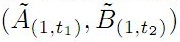where 0 t1,t21 are arbitrary. We first prove that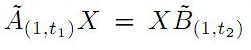if and only if AX=XB for all X, whenever (A,B) has the FP-property. We prove some similar results for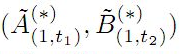instead of \$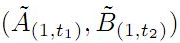as well. Also we introduce the sequence of generalized iterations of Aluthge transform of operators and express some results for this notion associated to the FP-property.

1: Paper Source PDF document

Paper's Title:

On Certain Classes of Harmonic Univalent Functions Based on Salagean Operator

Author(s):

G. Murugusundaramoorthy, Thomas Rosy, and B. A. Stephen

Department of Applied Mathematics and Informatics,
Department of Mathematics, Vellore Institute of Technology,
Deemed University, Vellore - 632014, India.
gmsmoorthy@yahoo.com

Department of Applied Mathematics and Informatics,
Department of Mathematics, Madras Christian College,
Chennai - 600059, India.
drthomasrosy@rediffmail.com

Abstract:

We define and investigate a class of complex-valued harmonic univalent functions of the form f = h + g using Salagean operator where h and g are analytic in the unit disc U = { z : |z| < 1 }. A necessary and sufficient coefficient conditions are given for functions in these classes. Furthermore, distortion theorems, inclusion relations, extreme points, convolution conditions and convex combinations for this family of harmonic functions are obtained.

1: Paper Source PDF document

Paper's Title:

Refinement Inequalities Among Symmetric Divergence Measures

Author(s):

Inder Jeet Taneja

Departamento de Matemática,
Universidade Federal de Santa Catarina, 88.040-900
Florianópolis, Sc, Brazil

URL: http://www.mtm.ufsc.br/~taneja

Abstract:

There are three classical divergence measures in the literature on information theory and statistics, namely, Jeffryes-Kullback-Leiber’s J-divergence, Sibson-Burbea-Rao’s Jensen- Shannon divegernce and Taneja’s arithemtic - geometric mean divergence. These bear an interesting relationship among each other and are based on logarithmic expressions. The divergence measures like Hellinger discrimination, symmetric χ2divergence, and triangular discrimination are not based on logarithmic expressions. These six divergence measures are symmetric with respect to probability distributions. In this paper some interesting inequalities among these symmetric divergence measures are studied. Refinements of these inequalities are also given. Some inequalities due to Dragomir et al.  are also improved.

1: Paper Source PDF document

Paper's Title:

Iterative Approximation of Common Fixed Points of a Finite Family of Asymptotically Hemi-contractive Type Mappings

Author(s):

Jui-Chi Huang

Center for General Education,
Northern Taiwan Institute of Science and Technology,
Peito, Taipei,
Taiwan, 11202, R.O.C.
juichi@ntist.edu.tw

Abstract:

In this paper, we prove that the sequence of the modified Ishikawa-Xu,
Ishikawa-Liu, Mann-Xu and Mann-Liu iterative types of a finite family of
asymptotically hemi-contractive type mappings converges strongly to a common
fixed point of the family in a real p-uniformly convex Banach space with
p>1. Our results improve and extend some recent results.

1: Paper Source PDF document

Paper's Title:

Generalizations of Hermite-Hadamard's Inequalities for Log-Convex Functions

Author(s):

Ai-Jun Li

School of Mathematics and Informatics, Henan Polytechnic University,
Jiaozuo City, Henan Province,
454010, China.
liaijun72@163.com

Abstract:

In this article, Hermite-Hadamard's inequalities are extended in terms of the weighted power mean and log-convex function. Several refinements, generalizations and related inequalities are obtained.

1: Paper Source PDF document

Paper's Title:

On a Method of Proving the Hyers-Ulam Stability of Functional Equations on Restricted Domains

Author(s):

Janusz Brzdęk

Department of Mathematics
Pedagogical University Podchor
ąźych 2,
30-084 Krak
ów,
Poland
jbrzdek@ap.krakow.pl

Abstract:

We show that generalizations of some (classical) results on the Hyers-Ulam stability of functional equations, in several variables, can be very easily derived from a simple result on stability of a functional equation in single variable

1: Paper Source PDF document

Paper's Title:

Harmonic Functions with Positive Real Part

Author(s):

Sďbel Yalçin

Uludag Universitesi,
Fen Edebiyat Fakultesi, Matematik Bolumu,
16059 Bursa,
Turkey

syalcin@uludag.edu.tr

Abstract:

In this paper, the class of harmonic functions f=h+{g} with positive real part and normalized by f(ζ )=1, (|ζ|<1) is studied, where h and g are analytic in U={z:|z|<1}. Some properties of this class are searched. Sharp coefficient relations are given for functions in this class. On the other hand, the author make use of Alexander integral transforms of certain analytic functions (which are starlike with respect to f(ζ)) with a view to investigating the construction of sense preserving, univalent and close to convex harmonic functions.

1: Paper Source PDF document

Paper's Title:

Some Identities for Ramanujan - Göllnitz - Gordon Continued fraction

Author(s):

M. S. Mahadeva Naika, B. N. Dharmendra and S. Chandan Kumar

Department of Mathematics,
Bangalore University,
Central College Campus,
Bangalore-560 001,
INDIA

msmnaika@rediffmail.com

chandan.s17@gmail.com

Department of Mathematics,
Maharani's Science College for Women,
J. L. B. Road, Mysore-570 001,
INDIA

dharmamath@rediffmail.com

Abstract:

In this paper, we obtain certain P--Q eta--function identities, using which we establish identities providing modular relations between Ramanujan-Göllnitz-Gordon continued fraction H(q) and H(q^n) for n= 2, 3, 4, 5, 7, 8, 9, 11, 13, 15, 17, 19, 23, 25, 29 and 55.

1: Paper Source PDF document

Paper's Title:

Hyponormal and K-Quasi-Hyponormal Operators On Semi-Hilbertian Spaces

Author(s):

Ould Ahmed Mahmoud Sid Ahmed and Abdelkader Benali

Mathematics Department,
College of Science,
Aljouf University,
Aljouf 2014,
Saudi Arabia.
E-mail: sididahmed@ju.edu.sa

Mathematics Department, Faculty of Science,
Hassiba Benbouali, University of Chlef,
B.P. 151 Hay Essalem, Chlef 02000,
Algeria.
E-mail: benali4848@gmail.com

Abstract:

Let H be a Hilbert space and let A be a positive bounded operator on H. The semi-inner product < u|v>A:=<Au|v>, u,v H induces a semi-norm || .||A on H. This makes H into a semi-Hilbertian space. In this paper we introduce the notions of hyponormalities and k-quasi-hyponormalities for operators on semi Hilbertian space (H,||.||A), based on the works that studied normal, isometry, unitary and partial isometries operators in these spaces. Also, we generalize some results which are already known for hyponormal and quasi-hyponormal operators. An operator T BA (H) is said to be (A, k)-quasi-hyponormal if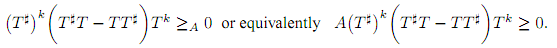1: Paper Source PDF document

Paper's Title:

Robust Error Analysis of Solutions to Nonlinear Volterra Integral Equation in Lp Spaces

Author(s):

Department of Mathematics, Faculty of Mathematics,
University of Sistan and Baluchestan, P.O. Box 98135-674, Zahedan,
Iran.
E-mail: h.baghani@gmail.com

Department of Mathematics, Faculty of Basic Sciences,
Birjand University of Technology, Birjand,
Iran.
E-mail: j.farrokhi@birjandut.ac.ir

Department of Mathematics and Computer Sciences,
Hakim Sabzevari University, P.O. Box 397, Sabzevar,
Iran.
E-mail: o.baghani@gmail.com

Abstract:

In this paper, we propose a novel strategy for proving an important inequality for a contraction integral equations. The obtained inequality allows us to express our iterative algorithm using a "for loop" rather than a "while loop". The main tool used in this paper is the fixed point theorem in the Lebesgue space. Also, a numerical example shows the efficiency and the accuracy of the proposed scheme.

1: Paper Source PDF document

Paper's Title:

The Dynamics of an Ebola Epidemic Model with Quarantine of Infectives

Author(s):

Eliab Horub Kweyunga

Department of Mathematics,
Kabale University,
P.O.Box 317, Kabale,
Uganda.

E-mail: hkweyunga@kab.ac.ug

Abstract:

The recurrent outbreaks of ebola in Africa present global health challenges. Ebola is a severe, very fatal disease with case fatality rates of up to 90%. In this paper, a theoretical deterministic model for ebola epidemic with quarantine of infectives is proposed and analyzed. The model exhibits two equilibria; the disease free and endemic equilibrium points. The basic reproduction number, R0, which is the main threshold, is obtained and the stability of the equilibrium points established. Using parameter values drawn from the 2014 West Africa ebola outbreak, a numerical simulation of the model is carried out. It is found that the dynamics of the model are completely determined by R0 and that a quarantine success rate of at least 70% is sufficient to contain the disease outbreak.

1: Paper Source PDF document

Paper's Title:

Some fixed point results in partial S-metric spaces

Author(s):

M. M. Rezaee, S. Sedghi, A. Mukheimer, K. Abodayeh, and Z. D. Mitrovic

Department of Mathematics, Qaemshahr Branch,
Iran.

Department of Mathematics, Qaemshahr Branch,
Iran.
E-mail: sedghi.gh@qaemiau.ac.ir

Department of Mathematics and General Sciences,
KSA.
E-mail: mukheimer@psu.edu.sa

Department of Mathematics and General Sciences,
KSA.
E-mail: kamal@psu.edu.sa

Nonlinear Analysis Research Group,
Faculty of Mathematics and Statistics,
Ton Duc Thang University, Ho Chi Minh City,
Vietnam.
E-mail: zoran.mitrovic@tdtu.edu.vn

Abstract:

We introduce in this article a new class of generalized metric spaces, called partial S-metric spaces. In addition, we also give some interesting results on fixed points in the partial S-metric spaces and some applications.

1: Paper Source PDF document

Paper's Title:

ψ(m,q)-Isometric Mappings on Metric Spaces

Author(s):

Sid Ahmed Ould Beinane, Sidi Hamidou Jah and Sid Ahmed Ould Ahmed Mahmoud

Mathematical Analysis and Applications, Mathematics Department, College of Science,
Jouf University,
Sakaka P.O.Box 2014,
Saudi Arabia.
E-mail: beinane06@gmail.com

Department of Mathematics, College of Science Qassim University,
P.O. Box 6640, Buraydah 51452,
Saudi Arabia.
E-mail: jahsiidi@yahoo.fr

Mathematical Analysis and Applications,
Mathematics Department, College of Science, Jouf University,
Sakaka P.O.Box 2014,
Saudi Arabia.
E-mail: sidahmed@ju.edu.sa, sidahmed.sidha@gmail.com

Abstract:

The concept of (m,p)-isometric operators on Banach space was extended to (m,q)-isometric mappings on general metric spaces in . This paper is devoted to define the concept of ψ(m, q)-isometric, which is the extension of A(m, p)-isometric operators on Banach spaces introduced in . Let T,ψ: (E,d) -> (E, d) be two mappings.
For some positive integer m and q (0,). T is said to be an ψ(m,q)-isometry, if for all  y,z E,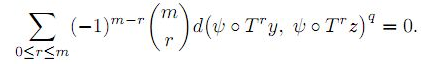1: Paper Source PDF document

Paper's Title:

Sharp Inequalities Between Hölder and Stolarsky Means of Two Positive Numbers

Author(s):

M. Bustos Gonzalez and A. I. Stan

The University of Iowa,
Department of Mathematics,
14 MacLean Hall,
Iowa City, Iowa,
USA.
E-mail: margarita-bustosgonzalez@uiowa.edu

The Ohio State University at Marion,
Department of Mathematics,
1465 Mount Vernon Avenue,
Marion, Ohio,
USA.
E-mail: stan.7@osu.edu

Abstract:

Given any index of the Stolarsky means, we find the greatest and least indexes of the H\"older means, such that for any two positive numbers, the Stolarsky mean with the given index is bounded from below and above by the Hölder means with those indexes, of the two positive numbers. Finally, we present a geometric application of this inequality involving the Fermat-Torricelli point of a triangle.

1: Paper Source PDF document

Paper's Title:

Generalized Von Neumann-Jordan Constant for Morrey Spaces and Small Morrey Spaces

Author(s):

H. Rahman and H. Gunawan

Department of Mathematics,
Islamic State University Maulana Malik Ibrahim Malang,
Jalan Gajayana No.50,
Indonesia.
E-mail: hairur@mat.uin-malang.ac.id

Analysis and Geometry Group,
Faculty of Mathematics and Natural Sciences,
Bandung Institute of Technology, Bandung 40132,
Indonesia.
E-mail: hgunawan@math.itb.ac.id
URL:  http://personal.fmipa.itb.ac.id/hgunawan/

Abstract:

In this paper we calculate some geometric constants for Morrey spaces and small Morrey spaces, namely generalized Von Neumann-Jordan constant, modified Von Neumann-Jordan constants, and Zbaganu constant. All these constants measure the uniformly nonsquareness of the spaces. We obtain that their values are the same as the value of Von Neumann-Jordan constant for Morrey spaces and small Morrey spaces.

Search and serve lasted 0 second(s).